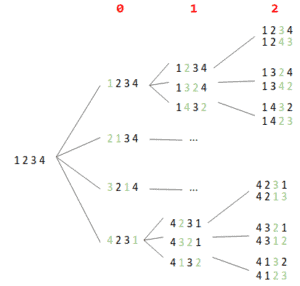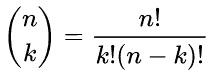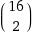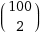### Get started with Spring 5 and Spring Boot 2, through the Learn Spring course:

>> CHECK OUT THE COURSE

## 1. Overview

In this tutorial, we’ll learn how to solve a few common combinatorial problems. They are most likely not very useful in an everyday job; however, they’re interesting from the algorithmic perspective. We may find them handy for testing purposes.

Keep in mind that there are many different approaches to solve these problems. We’ve tried to make the solutions presented easy to grasp.

## 2. Generating Permutations

First, let’s start with permutations. A permutation is an act of rearranging a sequence in such a way that it has a different order.

As we know from math, for a sequence of n elements, there are n! different permutations. n! is known as a factorial operation:

n! = 1 * 2 * … * n

So, for example, for a sequence [1, 2, 3] there are six permutations:

``````[1, 2, 3]

[1, 3, 2]

[2, 1, 3]

[2, 3, 1]

[3, 1, 2]

[3, 2, 1]``````

Factorial grows very fast — for a sequence of 10 elements, we have 3,628,800 different permutations! In this case, we talk about permuting sequences, where every single element is different.

### 2.1. Algorithm

It’s a good idea to think about generating permutations in a recursive manner. Let’s introduce the idea of the state. It will consist of two things: the current permutation and the index of the currently processed element.

The only work to do in such a state is to swap the element with every remaining one and perform a transition to a state with the modified sequence and the index increased by one.

Let’s illustrate with an example.

We want to generate all permutations for a sequence of four elements – [1, 2, 3, 4]. So, there will be 24 permutations. The illustration below presents the partial steps of the algorithm:Each node of the tree can be understood as a state. The red digits across the top indicate the index of the currently processed element. The green digits in the nodes illustrate swaps.

So, we start in the state [1, 2, 3, 4] with an index equal to zero. We swap the first element with each element – including the first, which swaps nothing – and move on to the next state.

Now, our desired permutations are located in the last column on the right.

### 2.2. Java Implementation

The algorithm written in Java is short:

``````private static void permutationsInternal(List<Integer> sequence, List<List<Integer>> results, int index) {
if (index == sequence.size() - 1) {
}

for (int i = index; i < sequence.size(); i++) {
swap(sequence, i, index);
permutationsInternal(sequence, permutations, index + 1);
swap(sequence, i, index);
}
}``````

Our function takes three parameters: the currently processed sequence, results (permutations), and the index of the element currently being processed.

The first thing to do is to check if we’ve reached the last element. If so, we add the sequence to the results list.

Then, in the for-loop, we perform a swap, do a recursive call to the method, and then swap the element back.

The last part is a little performance trick – we can operate on the same sequence object all the time without having to create a new sequence for every recursive call.

It might also be a good idea to hide the first recursive call under a facade method:

``````public static List<List<Integer>> generatePermutations(List<Integer> sequence) {
List<List<Integer>> permutations = new ArrayList<>();
permutationsInternal(sequence, permutations, 0);
return permutations;
}
``````

Keep in mind that the algorithm shown will work only for sequences of unique elements! Applying the same algorithm for sequences with recurring elements will give us repetitions.

## 3. Generating the Powerset of a Set

Another popular problem is generating the powerset of a set. Let’s start with the definition:

powerset (or power set) of set S is the set of all subsets of S including the empty set and S itself

So, for example, given a set [a, b, c], the powerset contains eight subsets:

``````[]

[a]

[b]

[c]

[a, b]

[a, c]

[b, c]

[a, b, c]``````

We know from math that, for a set containing n elements, the powerset should contain 2^n subsets. This number also grows rapidly, however not as fast as factorial.

### 3.1. Algorithm

This time, we’ll also think recursively. Now, our state will consist of two things: the index of the element currently being processed in a set and an accumulator.

We need to make a decision with two choices in each state: whether or not to put the current element in the accumulator. When our index reaches the end of the set, we have one possible subset. In such a way, we can generate every possible subset.

### 3.2. Java Implementation

Our algorithm written in Java is pretty readable:

``````private static void powersetInternal(
List<Character> set, List<List<Character>> powerset, List<Character> accumulator, int index) {
if (index == set.size()) {
} else {
powerSetInternal(set, powerset, accumulator, index + 1);
accumulator.remove(accumulator.size() - 1);
powerSetInternal(set, powerset, accumulator, index + 1);
}
}``````

Our function takes four parameters: a set for which we want to generate subsets, the resulting powerset, the accumulator, and the index of the currently processed element.

For simplicity, we keep our sets in lists. We want to have fast access to elements specified by index, which we can achieve it with List, but not with Set.

Additionally, a single element is represented by a single letter (Character class in Java).

First, we check if the index exceeds the set size. If it does, then we put the accumulator into the result set, otherwise we:

• put the currently considered element into the accumulator
• make a recursive call with incremented index and extended accumulator
• remove the last element from the accumulator, which we added previously
• do a call again with unchanged accumulator and the incremented index

Again, we hide the implementation with a facade method:

``````public static List<List<Character>> generatePowerset(List<Character> sequence) {
List<List<Character>> powerset = new ArrayList<>();
powerSetInternal(sequence, powerset, new ArrayList<>(), 0);
return powerset;
}``````

## 4. Generating Combinations

Now, it’s time to tackle combinations. We define it as follows:

k-combination of a set S is a subset of k distinct elements from S, where an order of items doesn’t matter

The number of k-combinations is described by the binomial coefficient:So, for example, for the set [a, b, c] we have three 2-combinations:

``````[a, b]

[a, c]

[b, c]``````

Combinations have many combinatorial usages and explanations. As an example, let’s say we have a football league consisting of 16 teams. How many different matches can we see?

The answer is, which evaluates to 120.

### 4.1. Algorithm

Conceptually, we’ll do something similar to the previous algorithm for powersets. We’ll have a recursive function, with state consisting of the index of the currently processed element and an accumulator.

Again, we’ve got the same decision for each state: Do we add the element to the accumulator? This time, though, we have an additional restriction – our accumulator can’t have more than k elements.

It’s worth noticing that the binomial coefficient doesn’t necessarily need to be a huge number. For example:is equal to 4,950, while

### 4.2. Java Implementation

For simplicity, we assume that elements in our set are integers.

Let’s take a look at the Java implementation of the algorithm:

``````private static void combinationsInternal(
List<Integer> inputSet, int k, List<List<Integer>> results, ArrayList<Integer> accumulator, int index) {
int needToAccumulate = k - accumulator.size();
int canAcculumate = inputSet.size() - index;

if (accumulator.size() == k) {
} else if (needToAccumulate <= canAcculumate) {
combinationsInternal(inputSet, k, results, accumulator, index + 1);
combinationsInternal(inputSet, k, results, accumulator, index + 1);
accumulator.remove(accumulator.size() - 1);
}
}``````

This time, our function has five parameters: an input set, k parameter, a result list, an accumulator, and the index of the currently processed element.

We start by defining helper variables:

• needToAccumulate – indicates how many more elements we need to add to our accumulator to get a proper combination
• canAcculumate – indicates how many more elements we can add to our accumulator

Now, we check if our accumulator size is equal to k. If so, then we can put the copied array into the results list.

In another case, if we still have enough elements in the remaining part of the set, we make two separate recursive calls: with and without the currently processed element being put into the accumulator. This part is analogous to how we generated the powerset earlier.

Of course, this method could’ve been written to work a little bit faster. For example, we could declare needToAccumulate and canAcculumate variables later. However, we are focused on readability.

Again, a facade method hides the implementation:

``````public static List<List<Integer>> combinations(List<Integer> inputSet, int k) {
List<List<Integer>> results = new ArrayList<>();
combinationsInternal(inputSet, k, results, new ArrayList<>(), 0);
return results;
}``````

## 5. Summary

In this article, we’ve discussed different combinatorial problems. Additionally, we’ve shown simple algorithms to solve them with implementations in Java. In some cases, these algorithms can help with unusual testing needs.

As usual, the complete source code, with tests, is available over on GitHub.

### Get started with Spring 5 and Spring Boot 2, through the Learn Spring course:

>> CHECK OUT THE COURSE